Courses

# Industrial Engineering - 2

## 20 Questions MCQ Test GATE Mechanical (ME) 2022 Mock Test Series | Industrial Engineering - 2

Description
This mock test of Industrial Engineering - 2 for GATE helps you for every GATE entrance exam. This contains 20 Multiple Choice Questions for GATE Industrial Engineering - 2 (mcq) to study with solutions a complete question bank. The solved questions answers in this Industrial Engineering - 2 quiz give you a good mix of easy questions and tough questions. GATE students definitely take this Industrial Engineering - 2 exercise for a better result in the exam. You can find other Industrial Engineering - 2 extra questions, long questions & short questions for GATE on EduRev as well by searching above.
QUESTION: 1

### Match the following numbers with the letters given below by using the cods given below: 1. A continuum describing the degree to which a system’s components may be separated and recombined. 2. The ability to move and change direction and position of the body quickly and effectively while under control. 3. The ability to withstand its variations in demand. 4. The product/part which can be usable even at its worst stage. p. Modularity q. Flexibility r. Robust s. Agility

Solution:
*Answer can only contain numeric values
QUESTION: 2

### An industrial machine tools manufacturer suppliers replacements from its inventory. For a particular part, the annual demand is expected to be 750 units. Machine setup costs are \$50, carrying costs are 25 percent per year, and the part is valued in inventory at \$35 each. The economic order quantity is __________ UNITS.

Solution: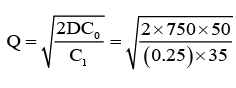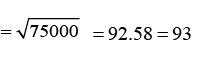QUESTION: 3

### The parts are processing on the machines launched into the system in the order of their arrivals

Solution:
QUESTION: 4

“The liner programming which starts with the optimal solution and infeasible solution, after some successive iteration, it will move to the optimal solution and the feasible” is

Solution:

The crux of the Dual simplex method is to start with the better than optimal and infeasible basic solution. The optimality and the feasibility conditions are designed to preserve the optimality and the feasibility of the basic solutions while moving the solution iterations toward feasibility.

QUESTION: 5

A dummy activity is used in PERT network to describe

Solution:
QUESTION: 6

Follow the symmetrical relation between primal and dual problems, assuming the primal to be a maximization problem,

(a) Number of variables in the primal equal to the number of constraints in the dual.

(b) Less than or equal to constraint in the primal equal to non-negative variable in the dual.

(c) Unrestricted variable in the primal equal to type constraint.

Among the above statements:

Solution:
QUESTION: 7

NC contouring is an example of

Solution:
QUESTION: 8

The 10-inch sine bar is used to measure the angularity of the taper on the shaft positioned between two bench centers. The difference in height (H 2 – H 1 ) over the buttons is measured using a Vernier height gauge and a dial test indicator. Calculate the included angle of the taper when H 2 = 6.122 inches and H 1 4.678 inches.

Solution: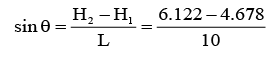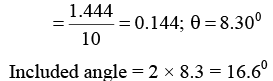*Answer can only contain numeric values
QUESTION: 9

In a 3-wire inspection of metric external thread of M16 × 2, the measurement over the wires were found to be 16.655 mm. Ten the effective pitch diameter of the thread is ……….. mm.

Solution: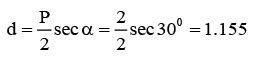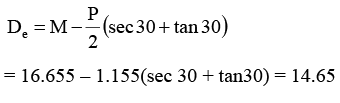QUESTION: 10

Match List – I with List – II and select the correct answers using the codes given below the lists: List – I

P. CNC
Q. DNS
R. FMS
S. Automatic lathe

List – II

1. Simultaneous control of several machine tools

2. Mass production of a single variety of parts

3. Flexible control of a single machine tool

4. Batch projection of a family of parts

Solution:
*Answer can only contain numeric values
QUESTION: 11

Demand for videotapes of a financial TB program varies uniformly between 500 and 900 copies. The distributor pays Rs. 4 per tape and realizes Rs. 18 income on each sale. Unsold tapes cannot be returned and have no salvage value. Then the optimum stocking level is ___________.

Solution: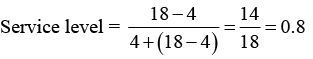Stocking level = 500 + 0.8 (900 – 500) = 500 + 320 = 820

QUESTION: 12

A repairman is to be hired by a company to repair machines whose break-down follow a Poisson process, with an average rate of four per hour. The cost of non-productive machine time is Rs. 90 per hour. The company has the option of choosing either a fast or slow repairman. The fast repairman (F) charges Rs. 70 per hour and will repair machines at an average rate of 7 per hour. While the slow repairman charges Rs. 50 per hour and repairs machines at an average rate of Rs. 6 per hour. Which repairman should be hired?

Solution:

Fast repairman Total wage = 70 × 8 = 560

Expected number of machinery in system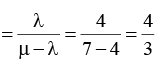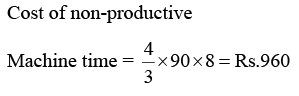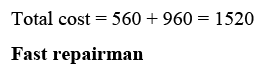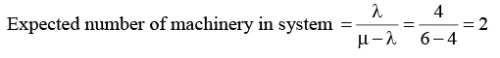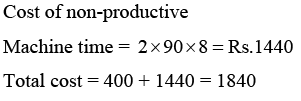QUESTION: 13

Demand averages 50 units per week with a standard deviation f 20 units per week; lead time is constant at 1 week (constant lead time with LT < order cycle). Then find the re-order point (ROP) for a service level of 98% from normal curve Z = 2.05 for 48%

Solution: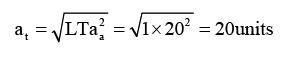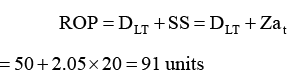QUESTION: 14

Consider the following statements: 1. ABC analysis is based on Pareto’s principal 2. MRP and MPS are the inputs for aggregate planning and control 3. Simulation can be used for inventory control 4. EOQ formula ignores variations in demand pattern

Solution:

ABC- Pareto → True

MRP, MPS – Aggregation → True

Simulation – Inventory → True

EOQ ignores variations in demand pattern → True

→ In the inventory A-class, B-class and C-class items were divided according to their nature. It was implemented by the scientist Advert Pareto an Italian based on 80:20 principle.

→ Material requirement planning in the Inp0ut for master-production schedule and the combination of MPS and MRP are inputs for aggregation production and planning.

→ In the inventory simulation can be done up to some extent.

→ Economic order quantity is very well applicable for the demands which are constant in demand.

QUESTION: 15

There are five jobs, each of which must go through the two machines A and B in the order AB. Processing times are given below:

Processing time (hours)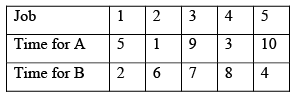By following the sequence procedure, calculate the total idle time for machine A and machine B respectively.

Solution: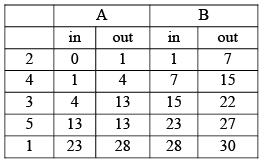Idle time for A → (28 to 30hrs ) = 2hrs Idle time for B → (0 − 1,22 − 23,27 − 28) = 3hrs

QUESTION: 16

Arrivals at a telephone booth are considered to be Poisson, with an average time of 10 minutes between one arrival and the next. The length of the phone call is assumed to be distributed exponentially, with mean time 3 minutes. Find the probability that an arrival finds that four persons are waiting for their turn.

Solution: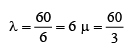customers/hair;  = 20 customers/hair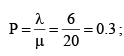four people waiting for their turn in the queue implies a total of 5 people in the system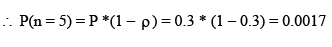QUESTION: 17

A farm machinery manufacture has an output rate of 320 hours per week and has measured the load on his shop as follows:

Work in Process:

Current Requirement – 960 hours

Long term orders – 320 hours

Then what is the manufacture lead time?

Solution: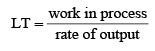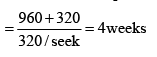QUESTION: 18

A company has 3 plants and four ware houses. The supply and demand in units and the corresponding transpiration costs are given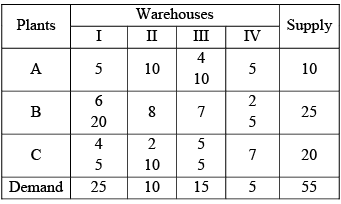Q.

Choose the correct statement

Solution:

The solution is not degenerate, because the number of occupied cells is not less than the required number of m + n – 1

Solution is optimal because all the ∆q's

The total cost involved is Rs. 235

QUESTION: 19

The parts are processing on the machines launched into the system in the order of their arrivals

Solution:
QUESTION: 20

If the cost for the route B-III is reduced form Rs. 7 to Rs. 6 per unit, what will be the total cost?

Solution:

If the cost per unit for the rate B-III equal to 6, it would have ∆ id = +1 and hence optimal solution obtained is x13 = 10; x 21 = 15; x 23 = 5; x 24 = 5; x 31 = 10 and x 32 = 10

Total cost = Rs. 230